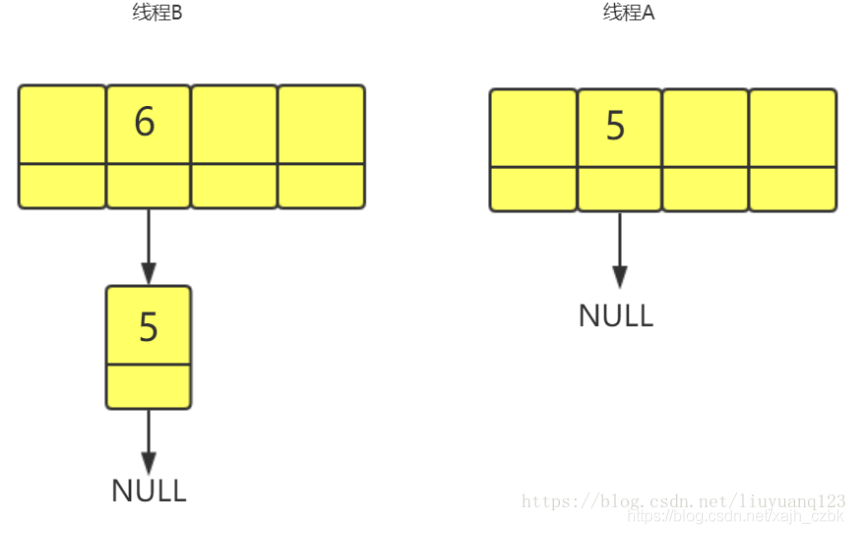# hashMap擴容時應該註意這些死循環問題

## hashMap死循環

1.原因： jdk1.7時使用頭插入法 ,1.8之後改成瞭尾插入法解決瞭這個問題

HashMap死循環問題圖解

```void resize(int newCapacity) {
Entry[] oldTable = table;
int oldCapacity = oldTable.length;
if (oldCapacity == MAXIMUM_CAPACITY) {
threshold = Integer.MAX_VALUE;
return;
}

Entry[] newTable = new Entry[newCapacity];
transfer(newTable, initHashSeedAsNeeded(newCapacity));
table = newTable;
threshold = (int)Math.min(newCapacity * loadFactor, MAXIMUM_CAPACITY + 1);
}
```

```void transfer(Entry[] newTable, boolean rehash) {
int newCapacity = newTable.length;
for (Entry<K,V> e : table) {
while(null != e) {
Entry<K,V> next = e.next;   ------（1）
if (rehash) {
e.hash = null == e.key ? 0 : hash(e.key);
}
int i = indexFor(e.hash, newCapacity);
e.next = newTable[i];
newTable[i] = e;
e = next;
}
}
}
``````e.next = newTable[i];
newTable[i] = e;``````e.next = newTable[i];
newTable[i] = e;``````e.next = newTable[i];
newTable[i] = e;```